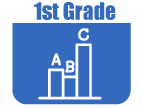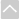Stand alone tasks are organized to support learning of content standards. These tasks can be used as initial instruction or to support students who are struggling with a particular topic.

Strand: MATHEMATICAL PRACTICES (1.MP)Strand: OPERATIONS AND ALGEBRAIC THINKING (1.OA)
Represent and solve problems involving addition and subtraction within 20 (Standards 1–2, 5–6).
• 20 Tickets
The purpose of the task is for students to add and subtract within 20 and represent complex addition problems with an equation to increase their understanding of and flexibility with the equals sign.
• Daisies in Vases
Given a scenario about daisies and vases, students must try to find as many ways as they can to put the daisies in the vases with the most in the large vase and the least in the smallest vase. They then must explain how they know they have found all the possibilities.
• Field Day Scarcity
The purpose of this task is for students to relate addition and subtraction problems to money in a context that introduces the concept of scarcity.
• Growing Bean Plants
This task adds some rigor to this common activity, by collecting actual growth data, providing practice for students in measuring and recording length measurements.
• Measuring Blocks
Have students work in pairs. Give each pair two blocks or strips to measure using unifix cubes.
• Sharing Markers
This task includes the three different problem types using the Take From context: result unknown, change unknown, and start unknown.
• The Pet Snake
Students are given 3 word problems about a pet snake. The task uses a continuous quantity for these examples of addition and subtraction.
• The Very Hungry Caterpillar
In this math lesson the teacher reads the book to the class and asks, "How many things do you think the caterpillar ate in this story?" The students take a minute to share their estimate with a partner. Next, the teacher reads The Very Hungry Caterpillar again. After each page, the teacher pauses so that the students can add counters or unifix cubes to the ten-frame to represent the number of things the caterpillar ate, and then write an equation on the dry-erase board connecting addition to the number of counters used.
Understand and apply properties of operations and the relationship between addition and subtraction (Standards 1.OA.3–4).
• Doubles?
Commentary on How 6 + 6 should be 12
Represent and solve problems involving addition and subtraction within 20 (Standards 1–2, 5–6).
• The Very Hungry Caterpillar
In this math lesson the teacher reads the book to the class and asks, "How many things do you think the caterpillar ate in this story?" The students take a minute to share their estimate with a partner. Next, the teacher reads The Very Hungry Caterpillar again. After each page, the teacher pauses so that the students can add counters or unifix cubes to the ten-frame to represent the number of things the caterpillar ate, and then write an equation on the dry-erase board connecting addition to the number of counters used.
Work with addition and subtraction equations (Standards 1.OA.7–8).
• 20 Tickets
The purpose of the task is for students to add and subtract within 20 and represent complex addition problems with an equation to increase their understanding of and flexibility with the equals sign.
• Equality Number Sentences
The purpose of this instructional task is for students to help students understand the meaning of the equal sign and to use it appropriately.
• Find the Missing Number
This task asks students to solve addition and subtraction equations with different structures so that they are able to see the connections between addition and subtraction more easily.
• Kiri's Mathematics Match Game - 1st Grade
In this activity students play a game in small groups that requires them to find sums or differences.
• The Very Hungry Caterpillar
In this math lesson the teacher reads the book to the class and asks, "How many things do you think the caterpillar ate in this story?" The students take a minute to share their estimate with a partner. Next, the teacher reads The Very Hungry Caterpillar again. After each page, the teacher pauses so that the students can add counters or unifix cubes to the ten-frame to represent the number of things the caterpillar ate, and then write an equation on the dry-erase board connecting addition to the number of counters used.
• Using lengths to represent equality
In this task students work in pairs and use Cuisenaire rods or paper strips cut to whole centimeter lengths. One student puts a few rods (or strips) end-to-end. The other student matches that length with a different combination of rods (or strips). When two different ways of making the same length are found, the students write a number sentence reflecting the equality.
• Valid Equalities?
The purpose of this task is to help broaden and deepen students understanding of the equals sign and equality. In this task, students must attend to the meaning of the equal sign by determining whether or not the left-hand expression and the right hand expression are equal. This task helps students attend to precision.Strand: NUMBER AND OPERATIONS IN BASE TEN (1.NBT)
Extend the counting sequence (Standard 1.NBT.1).
Understand place value (Standards 1.NBT.2–3).
• Ordering Numbers
The purpose of this task is to give students an opportunity to compare numbers less than 100 to benchmark numbers. Even though a number line is not explicitly given in the task, it is useful for students to list the numbers in the order they would appear on the number line; this allows them to focus on the relative ordering without worrying about the exact placement on the number line.
• The Very Hungry Caterpillar
In this math lesson the teacher reads the book to the class and asks, "How many things do you think the caterpillar ate in this story?" The students take a minute to share their estimate with a partner. Next, the teacher reads The Very Hungry Caterpillar again. After each page, the teacher pauses so that the students can add counters or unifix cubes to the ten-frame to represent the number of things the caterpillar ate, and then write an equation on the dry-erase board connecting addition to the number of counters used.
• Where Do I Go?
This activity is designed to be a short, repeatable activity to build student flexibility with the number sequence. Begin by randomly giving each student in the classroom one card from one of the sets you have made. Challenge the students to get themselves into order as quickly as they can. Once they are in order have the students read the cards beginning with the student with the smallest number to the largest.
Use place value understanding and properties of operations to add and subtract (Standards 1.NBT.4–6).
• Ford and Logan Add 45+36
This task was designed to give students opportunities to solve a problem and analyze other students solutions while working on adding two-digit numbers.
• Great Modeling Tasks in Three Acts - Thirsty Values
Students are going to discover how many CapriSun pouches are left on the table using place value.
• Number Square
This task describes an extended instructional sequence whose purpose is to use the 1-100 number square (also called a hundreds chart) to support understanding of adding and subtracting ten from a two-digit number.Strand: MEASUREMENT AND DATA (1.MD.)
Measure lengths indirectly and by iterating length units (Standards 1.MD.1–2).
• Growing Bean Plants
This task adds some rigor to this common activity, by collecting actual growth data, providing practice for students in measuring and recording length measurements.
• How Long?
In this task students use various items to measure, such as a large set of unifix or snap cubes and a recording sheet. The students work in pairs and choose an item to measure. They line up the cubes along the longest side of the item and then count and record the number on their sheet. They draw a picture of the item they measured.
• Measure Me!
The purpose of this task is for students to measure something that interests them (namely themselves) by laying multiple copies of a shorter object that represents the length unit end to end.
• Measuring Blocks
Have students work in pairs. Give each pair two blocks or strips to measure using unifix cubes.
Tell and write time (Standard 1.MD.3).
• Making a Clock
The purpose of this task is to introduce students to the concept of reading an analog clock.
Represent and interpret data (Standard 1.MD.4).
• Favorite Ice Cream Flavor
The purpose of this task is for students to represent and interpret categorical data. So this task could be used with advanced first graders or second graders just beginning to work with bar graphs.
• Weather Graph Data
The purpose of this task is for students to represent and interpret weather data, as well as answer questions about the data they have recorded.
Identify the value of coins (Standard 1.MD.5).Strand: GEOMETRY (1.G.)
Reason with shapes and their attributes (Standards 1.G.1–3).
• 3-D Shape Sort
The purpose of this task is to familiarize students with the idea of defining and non-defining attributes of geometric figures by using a collection of real objects or a set of cards with pictures of objects.
• All vs. Only some
The purpose of this task is for students to discuss and come to understand what constitute defining attributes for triangles, squares, and rectangles. Students start by looking for attributes shared by all the instances of a particular shape.
• Counting Squares
The purpose of this task is to give students an opportunity to compose and decompose squares. This is a challenging problem for first graders and it would be inappropriate to use it as an assessment.
• Equal Shares
This purpose of this task is for students to understand how to partition shapes into equal pieces.
• Grandfather Tang's Story
The purpose of this task is for students to compose two-dimensional shapes to create a composite shape using the 7 tangram pieces. The teacher reads Grandfather Tang's Story to the class noting the picture of the animal that is an outline of a shape that can be made with tangrams. After the class has read the story, the students choose animals from the story to make with the tangrams.The Online Core Resource pages are a collaborative project between the Utah State Board of Education and the Utah Education Network. If you would like to recommend a high quality resource, contact Trish French (Elementary) or Lindsey Henderson (Secondary). If you find inaccuracies or broken links contact resources@uen.org.